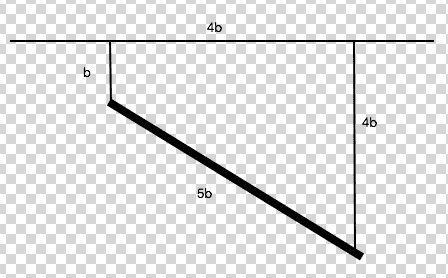# Calculate the Lagrangian of a coupled pendulum system

## Homework Statement

Calculate the Lagrangian of this set up:

Imagine having two ropes: They are both attached to the ceiling and have different lengths. One has length b and the other has length 4b. Say they are hooked to the ceiling a distance 4b apart. Now, the ropes are both hooked to a plank of mass M (uniform mass density) of length 5b. The rod can move in 3 dimensions. Ultimately, I am after the normal frequencies and normal modes of the system, but I think I can determine these if I can figure out this lagrangian## Homework Equations

$$\mathcal{L} = T-U$$

## The Attempt at a Solution

Well, Im not entirely sure how to go about this but my book suggests to use the coordinate $x$ for the longitudinal displacement of the rod and $y_1$ and $y_2$ as the sideways displacement of the rods two ends. Also, we are only assuming small displacements from equilibrium (so I think $\dot{z}$ is going to be zero)
Im not sure how to implement this choice of generalized coordinates.

Can anyone help me out? Also, I have never found a lagrangian for an extended object (its always been point masses in various systems)

Thanks in advance - btw I cannot find ANYTHING online that resembles a problem like this.

Orodruin
Staff Emeritus
Homework Helper
Gold Member
2021 Award
I suggest you go about it in the usual way. Write down the lagrangian of the system by expressing the kinetic and potential energies, then express those in terms of the generalised coordinate(s).

I've never written down the lagrangian of an extended object. I realize the K.E of the plank would be the K.E of its center of mass ($\frac{1}{2}m( \dot{x}^2+ \dot{y}^2)$ ) and probably some rotational K.E like $\frac{1}{2} I \omega^2$ but I dont know how the strings affect these terms...

Orodruin
Staff Emeritus
I've never written down the lagrangian of an extended object. I realize the K.E of the plank would be the K.E of its center of mass ($\frac{1}{2}m( \dot{x}^2+ \dot{y}^2)$ ) and probably some rotational K.E like $\frac{1}{2} I \omega^2$ but I dont know how the strings affect these terms...